# FOUR SQUARE QUESTIONS 1 4 Square Questions B

• Slides: 18FOUR SQUARE QUESTIONS 1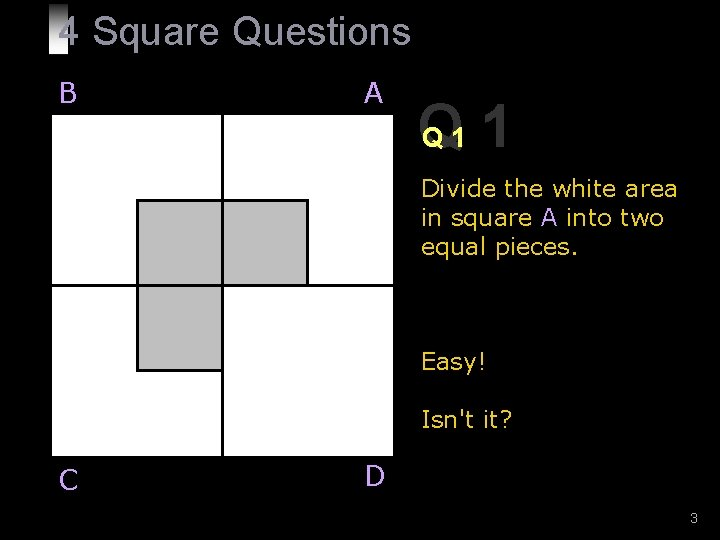4 Square Questions B A Q Q 1 1 Divide the white area in square A into two equal pieces. Easy! Isn't it? C D 3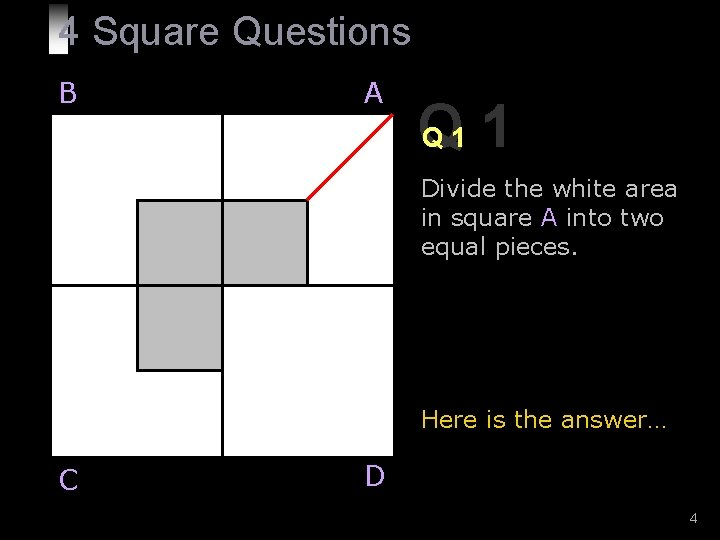4 Square Questions B A Q Q 1 1 Divide the white area in square A into two equal pieces. Here is the answer… C D 4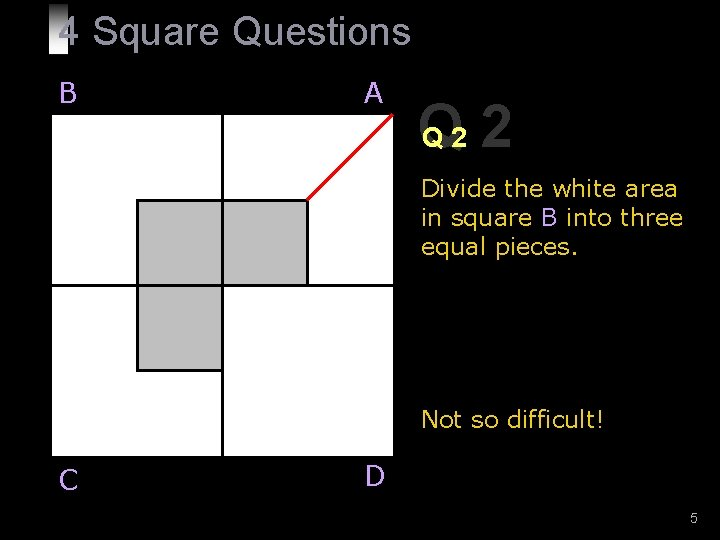4 Square Questions B A Q Q 2 2 Divide the white area in square B into three equal pieces. Not so difficult! C D 5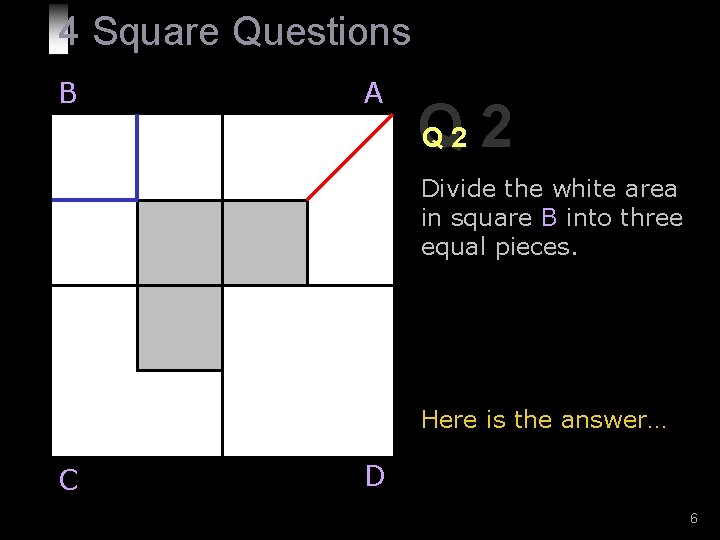4 Square Questions B A Q Q 2 2 Divide the white area in square B into three equal pieces. Here is the answer… C D 6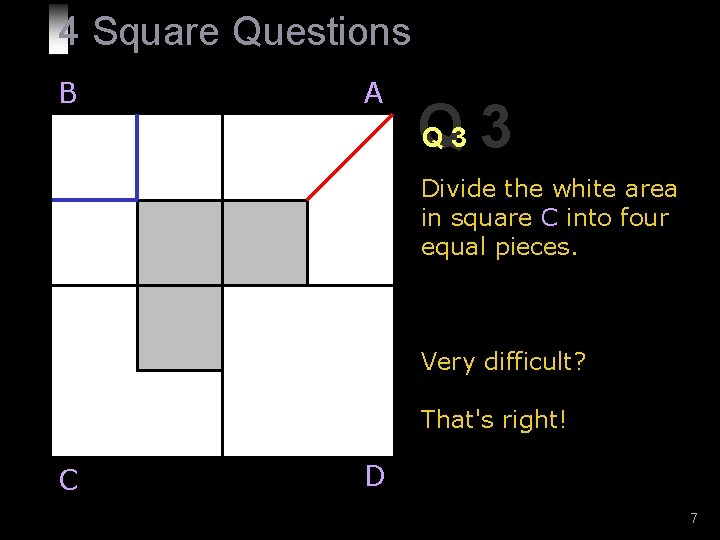4 Square Questions B A Q Q 3 3 Divide the white area in square C into four equal pieces. Very difficult? That's right! C D 7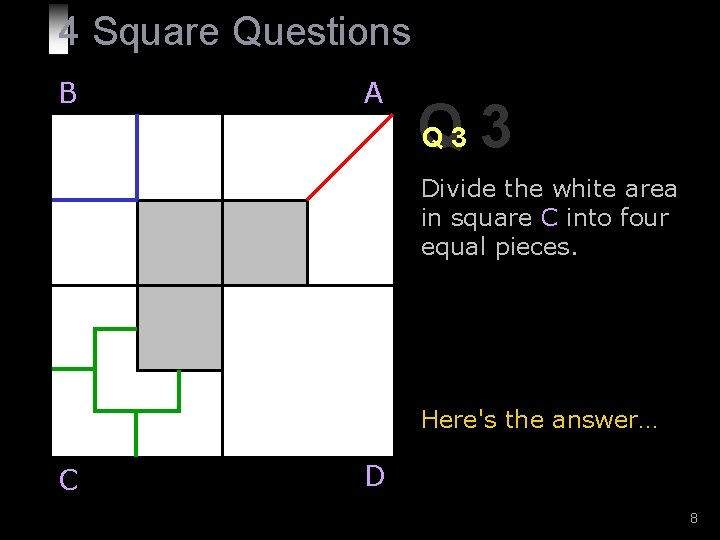4 Square Questions B A Q Q 3 3 Divide the white area in square C into four equal pieces. Here's the answer… C D 84 Square Questions B A Be ready! Here comes the last question… C D 9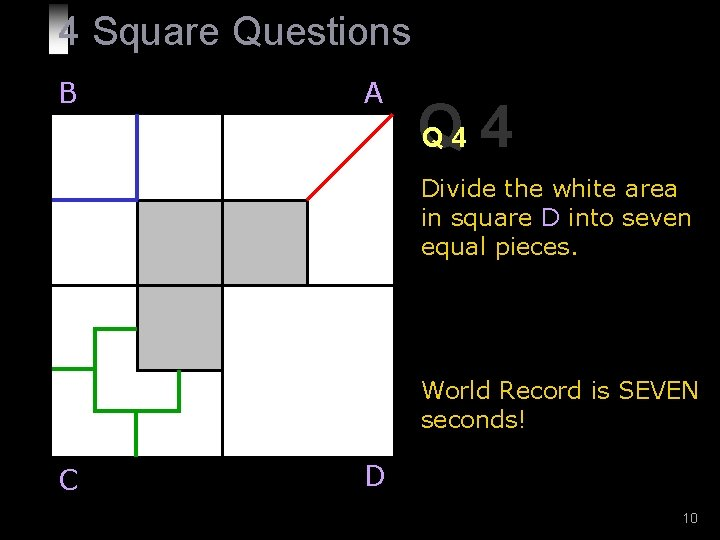4 Square Questions B A Q Q 4 4 Divide the white area in square D into seven equal pieces. World Record is SEVEN seconds! C D 10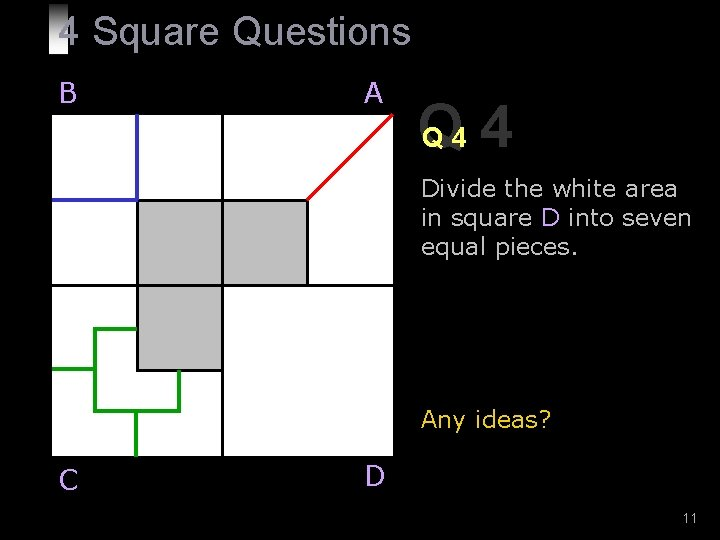4 Square Questions B A Q Q 4 4 Divide the white area in square D into seven equal pieces. Any ideas? C D 11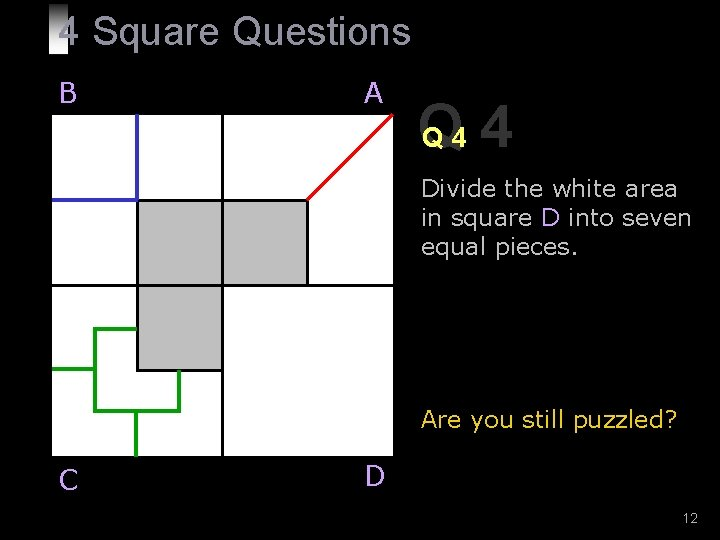4 Square Questions B A Q Q 4 4 Divide the white area in square D into seven equal pieces. Are you still puzzled? C D 12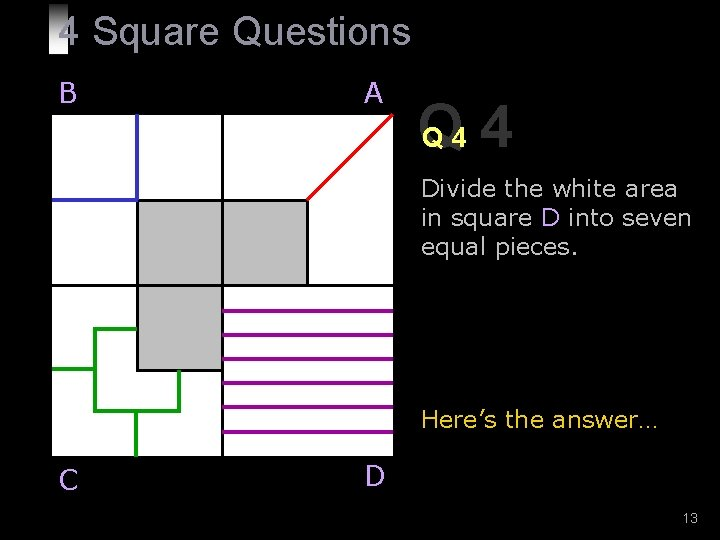4 Square Questions B A Q Q 4 4 Divide the white area in square D into seven equal pieces. Here’s the answer… C D 13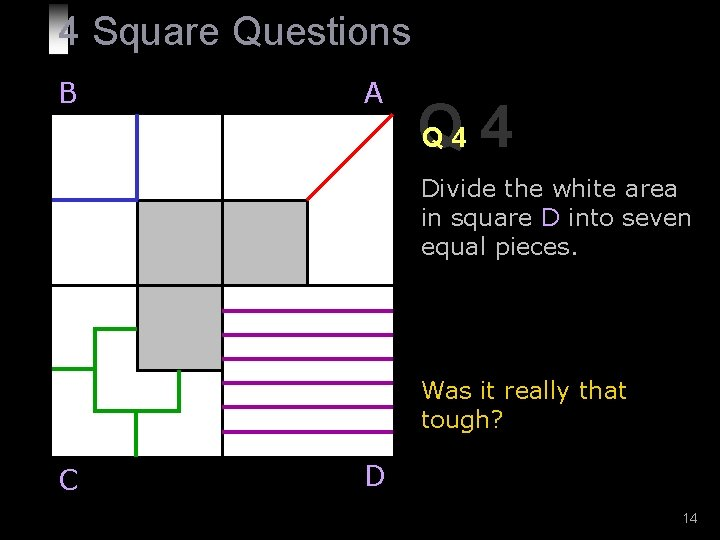4 Square Questions B A Q Q 4 4 Divide the white area in square D into seven equal pieces. Was it really that tough? C D 14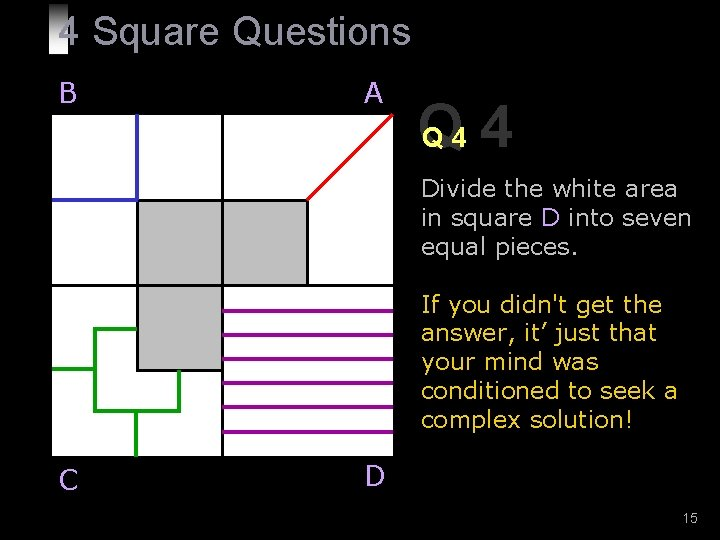4 Square Questions B A Q Q 4 4 Divide the white area in square D into seven equal pieces. If you didn't get the answer, it’ just that your mind was conditioned to seek a complex solution! C D 154 Square Questions Lessons learned: § Most of the time, our mind gets conditioned so much by the circumstances that we cannot see the obvious. § When we go to a specialist Doctor with a simple headache, diagnosis starts from migraine, upwards! § We assume that things are complicated, and we get entrapped in the ghost of its thoughts. § We carry on the same syndrome to our relationships and make them complicated. 16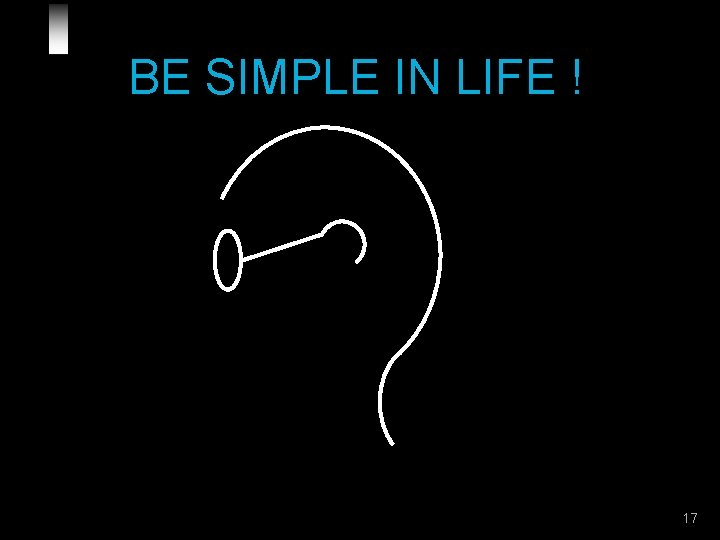BE SIMPLE IN LIFE ! 17joe-ks. com 18